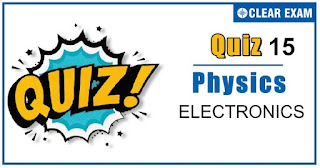## ELECTRONICS-QUIZ 15

As per analysis for previous years, it has been observed that students preparing for NEET find Physics out of all the sections to be complex to handle and the majority of them are not able to comprehend the reason behind it. This problem arises especially because these aspirants appearing for the examination are more inclined to have a keen interest in Biology due to their medical background. Furthermore, sections such as Physics are dominantly based on theories, laws, numerical in comparison to a section of Biology which is more of fact-based, life sciences, and includes substantial explanations. By using the table given below, you easily and directly access to the topics and respective links of MCQs. Moreover, to make learning smooth and efficient, all the questions come with their supportive solutions to make utilization of time even more productive. Students will be covered for all their studies as the topics are available from basics to even the most advanced. .

Q1. If ne and vd be the number of electrons and drift velocity in a semiconductor. When the temperature is increased
•  ne increases and vd decreases
•  ne decreases and vd increases
•  Both ne and vd increases
•  Both ne and vd decreases
Solution
(a) Because vd=i/((ne)eA)

Q2.To get an output 1 from the circuit shown in the figure, the input must be

•  A=0,B=1,C=0
•  A=1,B=0,C=0
•  A=1,B=0,C=1
•  A=1,B=1,C=0
Solution
(c) We can realize the gate circuit as Output of gate

1, Y1=A+B Output of gate 2, Y2=Y1∙C=(A+B)∙C (a) If A=0,B=1,C=0, then Y2=(0+1)∙0=1∙0=0 (b) If A=1,B=0,C=0, then Y2=(1+0)∙0=1∙0=0 (c) If A=1,B=0,C=1, then Y2=(1+0)∙1=1∙1=1 (d) If A=1,B=1,C=0, then Y2=(1+1)∙0=1∙0=0

Q3. When a p-n junction diode is reverse biased, then
•  No current flows
•  The depletion region is increased
•  The depletion region is reduced
•  The height of the potential barrier is reduced
Solution
(b) In reverse biasing, the applied voltage V on the n-side is positive and is negative on the p-side. The applied bias V and the barrier potential VBare in the same direction making the effective junction potential V+VB. As a result, the junction width will increase. The higher junction potential would restrict the flow of majority carriers to a much greater extent. However, such a field will favour the flow of minority carriers. So, reverse bias current will be due to the minority carriers only

Q4. When phosphorus and antimony are mixed in germanium, then
•  P-type semiconductor is formed
•  N-type semiconductor is formed
•  Both (a) and (b)
•  none of these
Solution
(b) Antimony and phosphorus both are pentavalent

Q5.Semiconductor material having fewer free electrons than pure germanium or silicon is
•  p-type
•  n-type
•  Both (a)and (b)
•  None of above
Solution
(a) In p-type semiconductor crystal has freely moving (positive) holes and an equal number of stationary negatively charged acceptor ions. Hence, p-type semiconductor material has fewer free electrons.

Q6. If nE and nH represent the number of free electrons and holes respectively in a semiconducting material,
then for n-type semiconducting material
•  nE < < nH
•  nE > > nH
• nE = nH
•  nE = nH = 0
Solution
(b) In an n-type semiconductor, majority charge carriers are electrons and minority charge carriers are holes. Therefore, nE >> nH

Q7.A 2V battery is connected across the points A and B as shown in the figure given below. Assuming that the resistance
of each diode is zero in forward bias and infinity in reverse bias, the current supplied by the battery when its
positive terminal is connected to A is

•  0.2 A
•  0.4 A
•  zero
•  0.1 A
Solution
(a)0.2 A

Q8.In a diode, when there is a saturation current, the plate resistance will be
•  Data insufficient
•  Zero
•  Some finite quantity
•  Infinite quantity
Solution
(d) We know that plate resistance is given by rp=δV/δI where δV is change in voltage and δI is change in current. Also, at saturation change in current is zero. ∴rp=δV/0=∞

Q9.The mutual characteristic curves of a triode are as shown in figure. The cut off voltage for the triode is

•  0 V
•  2 V
•  -4 V
•  6 V
Solution
c) From the graph it is clear that for Vg=-4V,ip=0, so cut off voltage is -4 volt

Q10. The output current versus time curve of a rectifier is shown in the figure. The average value of the output current in this case is

•  0
•  i0
•  2i0
•  i0
Solution
(c)2i0## Want to know more

Please fill in the details below:

## Latest NEET Articles\$type=three\$c=3\$author=hide\$comment=hide\$rm=hide\$date=hide\$snippet=hide

Name

ltr
item
BEST NEET COACHING CENTER | BEST IIT JEE COACHING INSTITUTE | BEST NEET & IIT JEE COACHING: ELECTRONICS-QUIZ 15
ELECTRONICS-QUIZ 15
https://1.bp.blogspot.com/-8D6w6EWbIew/X791zYRUWBI/AAAAAAAAEiA/ODinaJy36ugbqS8lRYJ5_twSRhcZwxfQwCLcBGAsYHQ/s320/Quiz%2BImage%2BTemplate%2B%252814%2529.jpg
https://1.bp.blogspot.com/-8D6w6EWbIew/X791zYRUWBI/AAAAAAAAEiA/ODinaJy36ugbqS8lRYJ5_twSRhcZwxfQwCLcBGAsYHQ/s72-c/Quiz%2BImage%2BTemplate%2B%252814%2529.jpg
BEST NEET COACHING CENTER | BEST IIT JEE COACHING INSTITUTE | BEST NEET & IIT JEE COACHING
https://www.cleariitmedical.com/2020/11/ELECTRONICS-QUIZ%2015.html
https://www.cleariitmedical.com/
https://www.cleariitmedical.com/
https://www.cleariitmedical.com/2020/11/ELECTRONICS-QUIZ%2015.html
true
7783647550433378923
UTF-8